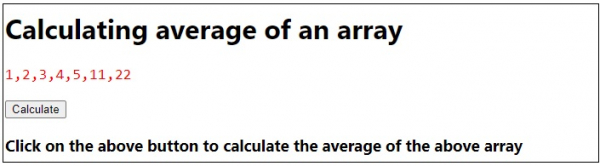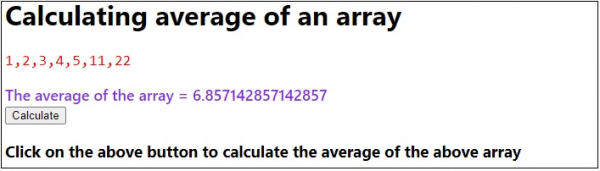# Calculating average of an array in JavaScript

Following is the code for calculating average of an array in JavaScript −

## Example

Live Demo

<!DOCTYPE html>
<html lang="en">
<meta charset="UTF-8" />
<meta name="viewport" content="width=device-width, initial-scale=1.0" />
<title>Document</title>
<style>
body {
font-family: "Segoe UI", Tahoma, Geneva, Verdana, sans-serif;
}
.result,
.sample {
font-size: 18px;
font-weight: 500;
color: blueviolet;
}
.sample {
color: red;
}
</style>
<body>
<h1>Calculating average of an array</h1>
<div><pre class="sample"></pre></div>
<div class="result"></div>
<button class="Btn">Check</button>
<h3>Click on the above button to see document state</h3>
<script>
let resEle = document.querySelector(".result");
let BtnEle = document.querySelector(".Btn");
let sampleEle = document.querySelector(".sample");
let arr = [1, 2, 3, 4, 5, 11, 22];
sampleEle.innerHTML = arr;
let sum = 0;
arr.forEach((item) => (sum += item));
resEle.innerHTML = "The average of the array = " + sum / arr.length;
});
</script>
</body>
</html>

## OutputOn clicking the ‘Calculate’ button −